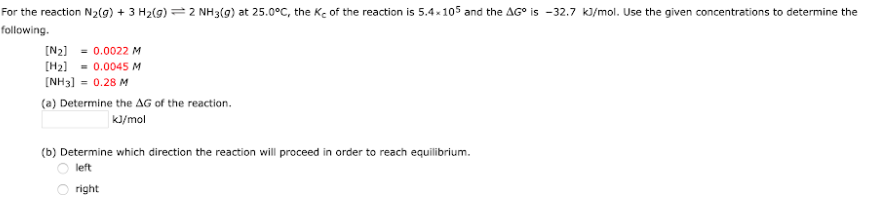# For the reaction N2(g) + 3 H2(g) <-> 2 NH3(g) at 25.0°C, the Kc of the reaction is 5.4 x 10^5 and the ΔG° is -32.7 kJ/mol. Use the given concentrations to determine the following. [N2] = 0.0022 M [H2] = 0.0045 M [NH3] = 0.28 M (a) Determine the ΔG of the reaction. (b) Determine which direction the reaction will proceed in order to reach equilibrium. left or right